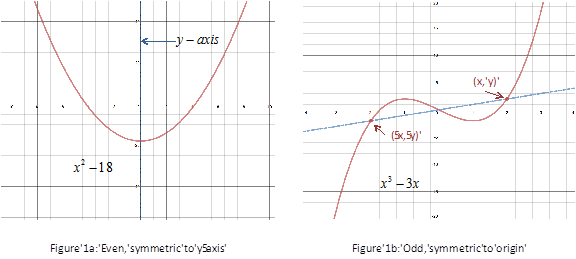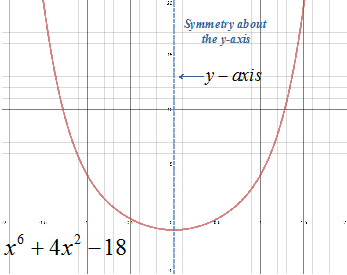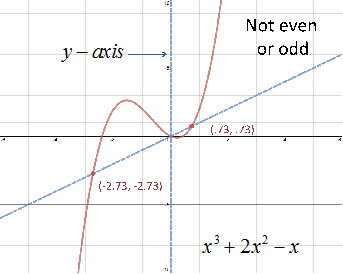# Even and Odd Functions

Some graphs exhibit symmetry. Graphs that have symmetry with respect to the y-axis are called even functions. Graphs the have symmetry with respect to the origin are called odd functions.

Look at the graphs of the two functions f(x) = x2 - 18 and g(x) = x3 - 3x. The function f(x) = x2 - 18 is symmetric with respect to the y-axis and is thus an even function. The function g(x) = x3 - 3x is symmetric about the origin and is thus an odd function.Stated another way, functions are even if changing x to -x does not change The value of the function.

EVEN FUNCTION:

f(x) = x2 - 18f(-x) = (-x)2 - 18 = x2 - 18

Since f(-x) = f(x) the function is even.

Functions are odd if changing x to -x negates the value of the function.

ODD FUNCTION:

f(x) = x3 - 3xf(-x) = (-x)3 - 3(-x) = -x3 + 3x = -(x3 - 3x)

Since f(-x) = -f(x) the function is odd.

A function can be even, odd or neither even nor odd. To determine if a function has even or odd symmetry use the following guidelines.

GUIDELINES TO DETERMINE EVEN OR ODD SYMMETRY

1. Replace the f(x) with f(-x) and simplify the function.

2. Compare the results of step 1 to f(x) and -f(x).

3. Determine if the function is even, odd or neither

a. If f(-x) results in the same value as f(x), the function is even.

b. If f(-x) results in the same value as -f(x), the function is odd.

c. If f(-x) did not result in step a or b, the function is neither even nor odd.

Let's look at a couple examples.

Example 1: Determine if the function f(x) = x6 + 4x2 - 1 is even, odd or neither then graph the function and describe the symmetry.
 Step 1: Replace f(x) with f(-x) and simplify the function. Original function: f(x) = x6 + 4x2 - 1 Substitute x with -x: f(-x) = (-x)6 + 4(-x)2 - 1 Simplify: f(-x) = x6 + 4x2 - 1 Step 2: Compare f(-x) to f(x) and -f(x). f(-x) = x6 + 4x2 - 1 f(x) = x6 + 4x2 - 1 Compare f(-x) to f(x): ${x}^{6}+4{x}^{2}-1{=}{x}^{6}+4{x}^{2}-1$ Since f(-x) to f(x) there is no need to compare f(-x) to -f(x) because the function cannot be both. Step 3: Determine if the function is even, odd, or neither. Since f(-x) = f(x) the function is even and has symmetry about the y-axis. Step 4: Graph the functionExample 2: Determine if the function f(x) = x3 + 2x2 - x is even, odd or neither then graph the function and descried the symmetry.
 Step 1: Replace f(x) with f(-x) and simplify the function. Original function: f(x) = x3 + 2x2 - x Substitute x with -x: f(-x) = (-x)3 + 2(-x)2 - (-x) Simplify: f(-x) = -x3 + 2x2 + x Step 2: Compare f(-x) to f(x) and -f(x). f(-x) = -(x3 - 2x2 - x) f(x) = x3 + 2x2 - x -f(x) = -(x3 + 2x2 - x) Compare f(-x) to f(x): $-\left({x}^{3}-2{x}^{2}-x\right){\ne }{x}^{3}+2{x}^{2}-x$ Compare f(-x) to -f(x): $-\left({x}^{3}-2{x}^{2}-x\right){\ne }-\left({x}^{3}+2{x}^{2}-x\right)$ Step 3: Determine if the function is even, odd, or neither. Since f(-x) does not equal f(x) or -f(x) the function is neither even nor odd. Step 4: Graph the functionRelated Links: Math algebra Function Transformations Rolle's Theorem Calculus Topics

To link to this Even and Odd Functions page, copy the following code to your site: